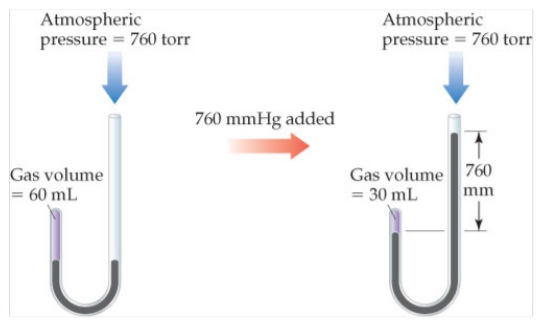# Problem: Boyle's experiment relating pressure and volume for a gas. (a) What is the total pressure on the gas after the 760 mm Hg has been added?

###### FREE Expert Solution

Recall that the ideal gas law is:

$\overline{){\mathbf{PV}}{\mathbf{=}}{\mathbf{nRT}}}$

96% (303 ratings)###### Problem Details

Boyle's experiment relating pressure and volume for a gas.(a) What is the total pressure on the gas after the 760 mm Hg has been added?